## 4.13Axisymmetric (wedge) condition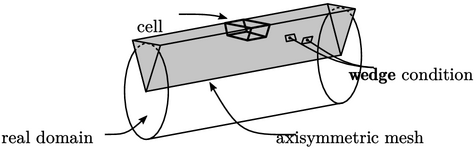There are some ﬂuid ﬂow problems for which the geometry is axisymmetric. Assuming the ﬂow solution is axisymmetric, i.e. ﬁelds do not change in the circumferential direction, the computational mesh can be formed of a wedge-shaped slice of the ﬂow geometry.

This type of mesh for axisymmetric solution contains one cell across the circumferential direction, which reduces the number of cells to two dimensions in the axial and radial directions.

This approach to axisymmetric solution introduces a geometric error due the faces normal to the radial direction being ﬂat. This error reduces with decreasing wedge angle; in practice, the error can be considered negligible for an angle of 1.

The wedge boundary condition is applied to the two sloping side patches. It transforms cell values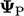to the patch faces using a rotational transformation tensor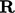by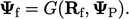(4.20)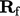deﬁnes a rotation between the unit vector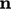in the circumferential direction at the cell centre and the unit face normal vector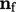by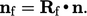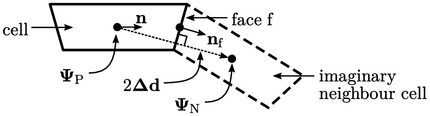The wedge condition uses the general transform framework of Sec. 4.11 , with the explicit value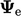calculated using current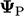from Eq. (4.20). The explicit gradient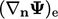is the boundary gradient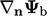calculated from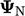in an imaginary neighbour cell by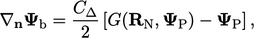(4.21)
where the rotation between cell centres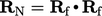.

The factor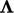is chosen to minimise the gradient boundary coeﬃcients (see Sec. 4.11 ). The vector factor is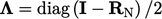where “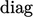” is deﬁned in Sec. 4.12 .

For a tensor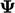, the factor is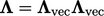, where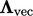are the coeﬃcients for a vector, as described for the symmetry condition in Sec. 4.12 .

### Rotation tensor

The rotation tensor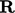between two unit vectors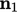and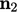can be calculated using the Euler-Rodrigues rotation formula,6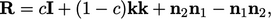where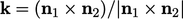and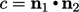.
6Benjamin Olinde Rodrigues, De l’attraction des sphéroïdes, 1815.

Notes on CFD: General Principles - 4.13 Axisymmetric (wedge) condition